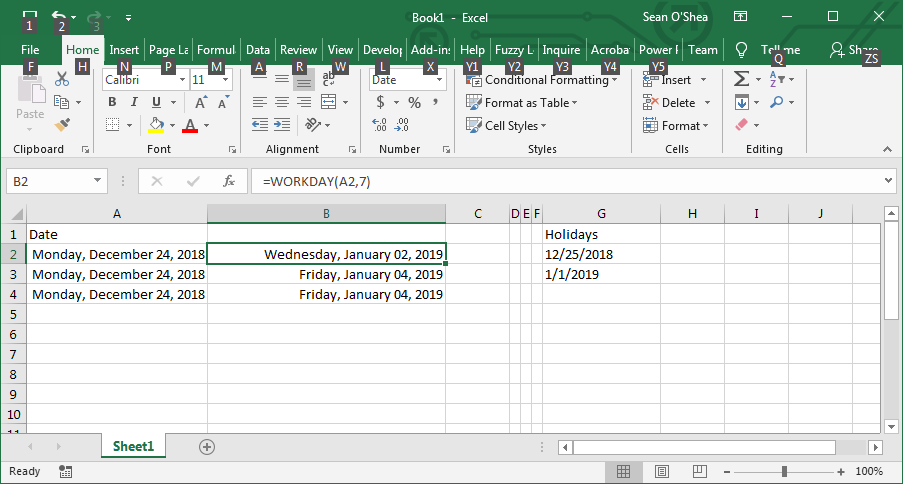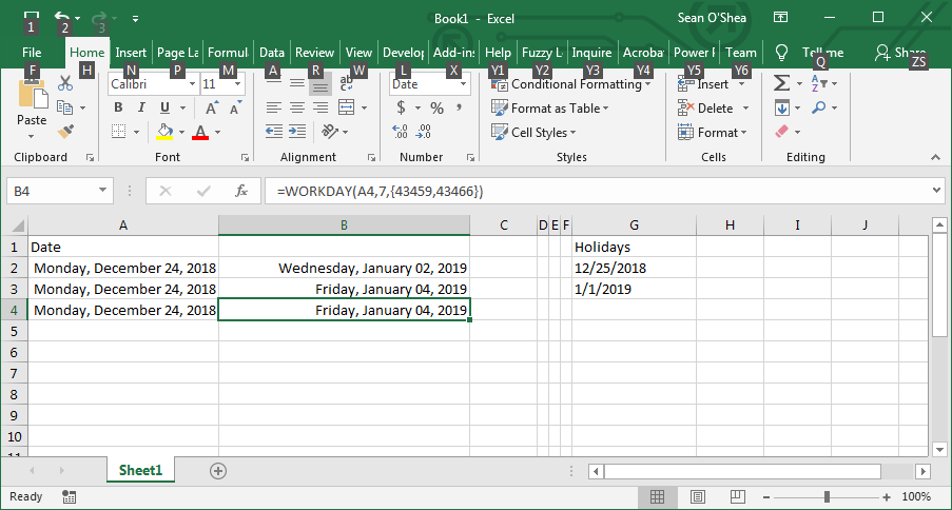top of pageSearch

# Workdays in Excel

The WORKDAYS formula can be used in Excel to find what date is a given number of workdays from a set day, including that day. So in this example, the formula: =WORKDAY(A2,7)

. . . finds that seven days including from and after 12/24/2018 it will be January 2, 2019.The WORKDAYS formula can also take account of holidays. In this example the holidays are listed in the range G2:G3. The formula: =WORKDAY(A3,7,G2:G3)

. . . finds that 7 workdays are in the period from 12/24/2018 through January 4, 2018, excluding the Christmas and New Year's Day holidays.The holidays can be entered as serial numbers. 43459 is the serial number for 12/25/2018 and 43466 is the serial number for 1/1/2019.The NETWORKDAYS formula will find the number of workdays in a given range. So in this example the formula:

=NETWORKDAYS(A2,B2)

. . .finds that there are 8 workdays in the range from 12/24/2018 to 1/2/2019.With the NETWORKDAYS formula, don't enter the actual dates in the formula, but make reference to the cells which contain the dates, unless you use the serial number format.

=NETWORKDAYS(43458,43467)It is also possible to insert dates into a formula under Formula . . . Date & Time . . . DATEEnter the values and Excel will generate the correct serial number.A new formula in this format:

=NETWORKDAYS(DATE(2018,12,24),DATE(2019,1,2))

. . . will be generated.bottom of page